Courses

# Design Parameters of Journal Bearing Mechanical Engineering Notes | EduRev

## Mechanical Engineering : Design Parameters of Journal Bearing Mechanical Engineering Notes | EduRev

The document Design Parameters of Journal Bearing Mechanical Engineering Notes | EduRev is a part of the Mechanical Engineering Course Machine Design.
All you need of Mechanical Engineering at this link: Mechanical Engineering

Design parameters of journal bearing

The first step for journal bearing design is determination of bearing pressure for the given design parameters,

Operating conditions (temperature, speed and load)
Geometrical parameters (length and diameter)
Type of lubricant (viscosity)

The design parameters, mentioned above, are to be selected for initiation of the design. The bearing pressure is known from the given load capacity and preliminary choice of bearing dimensions. After the bearing pressure is determined, a check for proper selection of design zone is required. The selection of design zone is explained below.

Selection of design zone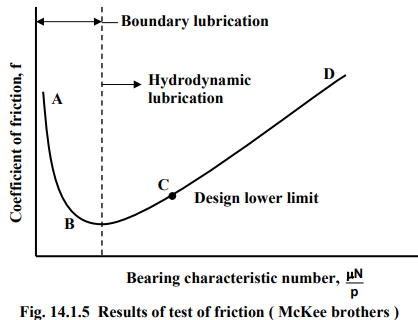The Fig. 14.1.5 shows the results of test of friction by McKee brothers. Figure shows a plot of variation of coefficient of friction with bearing characteristic number. Bearing characteristic number is defined as, Bearing characteristic number = μN/P

It is a non-dimensional number, where μ is the viscosity, N is the speed of the bearing and p is the pressure given by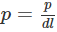d and l being diameter and length of the journal respectively.

The plot shows that from B with the increase in bearing characteristic number the friction increases and from B to A with reduction in bearing characteristic number the friction again increases. So B is the limit and the zone between A to B is known as boundary lubrication or sometimes termed as imperfect lubrication. Imperfect lubrication means that metal – metal contact is possible or some form of oiliness will be present. The portion from B to D is known as the hydrodynamic lubrication .The calculated value of bearing characteristic number should be somewhere in the zone of C to D. This zone is characterized as design zone.

For any operating point between C and D due to fluid friction certain amount of temperature generation takes place. Due to the rise in temperature the viscosity of the lubricant will decrease, thereby, the bearing characteristic number also decreases. Hence, the operating point will shift towards C, resulting in lowering of the friction and the temperature. As a consequence, the viscosity will again increase and will pull the bearing characteristic number towards the initial operating point. Thus a self control phenomenon always exists. For this reason the design zone is considered between C and D. The lower limit of design zone is roughly five times the value at B. On the contrary, if the bearing characteristic number decreases beyond B then friction goes on increasing and temperature also increases and the operation becomes unstable.

Therefore, it is observed that, bearing characteristic number controls the design of journal bearing and it is dependent of design parameters like, operating conditions (temperature, speed and load), geometrical parameters (length and diameter) and viscosity of the lubricant.

Methods for journal bearing design

Broadly there are two methods for journal bearing design, they are,

First Method: developed by M. D. Hersey

Second Method: developed by A. A. Raimondi and J. Boyd

Method developed by M. D. Hersey

This method is based on dimensional analysis, applied to an infinitely long bearing. Analysis incorporates a side-flow correction factor obtained from the experiment of S. A. McKee and T. R. McKee (McKee Brothers).

McKee equation for coefficient of friction, for full bearing is given by,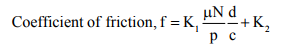(14.14)

Where,

p : pressure on bearing (projected area) = P /Ld
L : length of bearing
d : diameter of journal
N : speed of the journal
μ : absolute viscosity of the lubricant
c : difference bush and journal diameter
K2 : side-flow factor = 0.002 for (L/d) 0.75-2.8

The constant Kis dependent on the system of units. For example,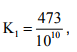when μ is in centipoise, p is in psi , N is in rpm and d and c in inches.

The steps to be followed are,

Basic design parameters are provided by the designer from the operating conditions. These are,

Bearing load (P)

Journal diameter (d)

Journal speed (N)

Depending upon type of application, selected design parameters are obtained from a design handbook, these are,

L/d ratio

Bearing pressure(p)

c/d ratio

Proper lubricant and an operating temperature

The heat generation in the bearing is given by,

Hg =  fPv where, v is the rubbing velocity

The heat dissipation is given by,

Hd = KA(tb - ta)

where,

A =  projected bearing area

K= heat dissipation coefficient

tb= bearing surface temperature

ta=  temperature of the surrounding

Next steps are as follows,

Value of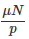should be within the design zone

Equation (14.1.7) is used to compute f

Heat generation and heat dissipation are computed to check for thermal equilibrium.

Iteration with selected parameters is required if thermal equilibrium is not established.

Provision for external cooling is required if it is difficult to achieve thermal equilibrium. The method described here is relatively old. The second method is more popular and is described below.

Method developed by A. A. Raimondi and J. Boyd

This method is based on hydrodynamic theory. The Reynolds equation (14.1.1) does not have any general solution. Assuming no side flow, Sommerfeld (1904) proposed a solution and defined a parameter, known as Sommerfeld number, given as,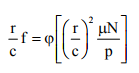(14.1.5)

where, φ = A functional relationship, for different types of bearings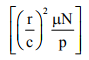= Sommerfeld number, S (dimensionless)

The Sommerfeld number is helpful to the designers, because it includes design parameters; bearing dimensions r and c , friction f , viscosity μ, speed of rotation N and bearing pressure p. But it does not include the bearing arc. Therefore the functional relationship can be obtained for bearings with different arcs, say 360ο , 60ο etc.

Raimondi and Boyd (1958) gave a methodology for computer–aided solution of Reynolds equation using an iterative technique. For L/d ratios of 1, 1:2 and 1:4 and for bearing angles of 3600 to 600 extensive design data are available. Charts have been prepared by Raimondi and Boyd for various design parameters, in dimensionless form, are plotted with respect to Sommerfeld number.

All these charts are for 600-600  bearings.

Materials for bearing

The common materials used for bearings are listed below.

Lead based babbits : around 85 % Lead; rest are tin, antimony and copper (pressure rating not exceeding 14MPa)

Tin based babbits : around 90% tin; rest are copper, antimony and lead (pressure rating not exceeding 14MPa)

Phosphor bronze : major composition copper; rest is tin, lead, phosphorus (pressure rating not exceeding 14MPa)

Gun metal : major composition copper; rest is tin and zinc (pressure rating not exceeding 10MPa)

Cast iron : pressure rating not exceeding 3.5 MPa

Other materials commonly used are, silver, carbon-graphite, teflon etc.

Offer running on EduRev: Apply code STAYHOME200 to get INR 200 off on our premium plan EduRev Infinity!

## Machine Design

57 videos|71 docs|102 tests

,

,

,

,

,

,

,

,

,

,

,

,

,

,

,

,

,

,

,

,

,

;